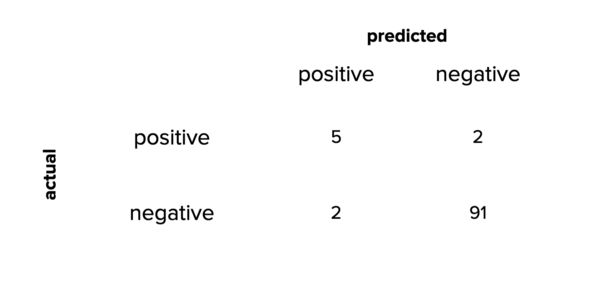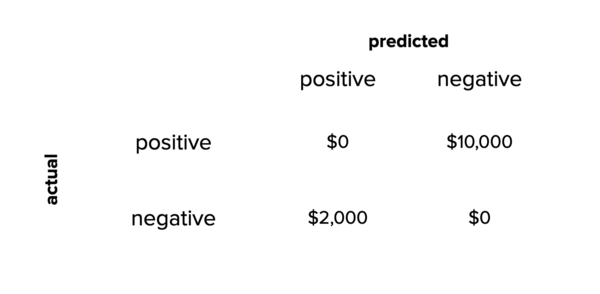# Cutting costs

 Cutting costs By Santiago • Issue #12 • View online Saving money is always a good reason to listen carefully, and cost sensitivity is one of my go-to tricks to get a little bit of attention.If you haven’t heard of it, keep reading. I promise you’ll get a lot of mileage out of it.Enjoy!
 A short introduction to Cost-Sensitive Learning
 Think of a factory. Now imagine a machine learning model that looks at a picture of a mechanical component and predicts whether it’s about to break.Don’t worry about how the model does this. Let’s focus on the results instead.Imagine we run 100 pictures through the model, and we get the following results: 7 components are about to break. These would be “Positive” results from the model. 93 components are fine. These would be “Negative” results from the model. There’s a lot we can do with this information.The best picture I could find related to "mechanical components" in a factory.
 The confusion matrixBefore anything else, it’s always a good idea for a person to manually review each of the model results so we can better understand how it’s working. Let’s say these are the results we get: Out of the 7 positive model results, 5 of those are about to break, but 2 are fine. Out of the 93 negative results, 91 are fine, but the other 2 are not working as expected. We can represent this information in a confusion matrix. This is what it’d look like:The confusion matrix for our hypothetical example.
 From here, we can easily compute all sort of metrics: The accuracy of our model is 96%. The precision is 71%. The recall is 71%. This is cool and all, but we can make things more interesting.Not every mistake is the sameThe model is currently making 4 mistakes: It’s classifying 2 components that are about to break as being fine. We call these false negatives. It’s classifying 2 components that are working correctly as positive. We call these false positives. What would happen if we ask the factory to assign a cost to each one of these mistakes?Let’s make a couple more assumptions: Every false-negative mistake means that the factory won’t replace a component before it breaks, so there will be downtime. Every time this happens, it will cost \$5,000. Every false-positive mistake means that the factory will have to send a technician to replace a component that isn’t broken. It’ll waste time, plus the shipping and storage costs of a replacement that is not needed. Every time this happens, it will cost \$1,000. We can use this information to create a new confusion matrix, but this time using the total cost of every mistake:The same confusion matrix, but looking at cost instead of number of mistakes.
 It should be clear now that we want our model to minimize the number of false-negative mistakes because they are 5 times more expensive than false-positive mistakes. In other words, we want to maximize the recall of this model.But there’s more!Can we use the cost of each mistake to optimize the results of the model?Changing the result of the modelWe have a binary classification model.Let’s imagine that the model returns a probability score attached to each answer. Whenever it says that a component is about to break, it also returns how confident on that answer it is. The complement of that probability corresponds to the opposite answer.Can we use this probability to optimize the model?Let’s imagine an example where the model looks at a picture and decides the result is “Negative” with a 57% probability. This would be the correct answer if we don’t want to do anything else, but we can get fancier than that: If the model returns “Negative,” there’s a 43% chance the result is actually “Positive,” so the potential cost of returning “Negative” is 0.43 × \$5,000 = \$2,150. If the model returns “Positive,” there’s a 57% chance the result is actually “Negative,” so the potential cost of returning “Positive” is 0.57 × \$1,000 = \$570. So yes, the model thinks the result is “Negative,” but we are better off returning “Positive” if we want to reduce the potential costs of running it.Pretty cool, huh?Final wordsI took you down a long rabbit hole to illustrate how things work, but a simpler way to have the model make final decisions is by adjusting the confidence threshold according to the cost of every mistake.This technique is part of a family of approaches known as Cost-Sensitive Learning, and it’s instrumental in real-world scenarios where money is an important factor. Here is an article going deeper into cost-sensitive learning, specifically for imbalanced datasets. Spend some time thinking about this, and I promise it will change the way you approach many of the problems you’ll face out there.
 Don't talk and drive
 Did you enjoy this issue?I'll send you an email whenever I publish something important. I promise you don't want to miss this.